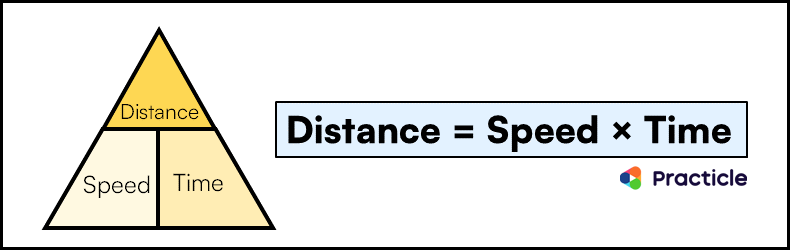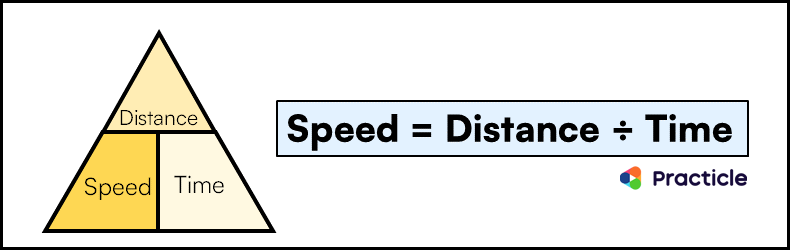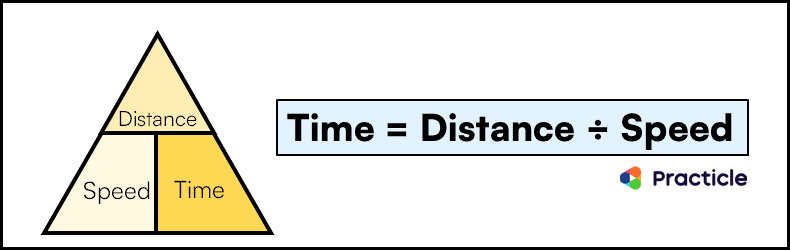PSLE Math simplified

# Solve speed questions easily

When it comes to preparing for PSLE math, speed always comes up as one of the most challenging Primary 6 topics according to most students and parents. In this simple Practicle guide on speed, let’s look at the different kind of speed questions that can appear in your PSLE math paper and learn how to solve them.

### The only speed formula you’ll need

Let’s start with the Speed questions you’ll see in Paper 1. These Math problems usually test you on your basics. This means that you can solve them with the Distance Speed Time formula, the Speed Time Distance formula or the Time Speed Distance formula.

Let’s look at each ingredient of these formulas:

• Distance – Distance measures how far the object travels
• Speed – Speed measures how fast an object travels from one place to another. (usually in m/s or km/h)
• Time – Time is how long the object takes to travelIf you are driving a car at a speed of 60 km/h, this means that the car is going to travel 60 km every hour. When you drive it for 2 hours, you would have covered a total distance of 60 km/h x 2 h which is 120 km. This is why distance = speed * time.

Knowing this relationship between speed, time and distance is key to solving any speed question.

### Speed Questions in Math Paper 1

Now let’s see how a speed question in your PSLE Paper 1 might look like.

These speed questions will provide you with 2 known values out of the 3 ingredients most of the time. The missing third value that you are asked to solve can either be the speed, time or distance.

For example, you might be asked to solve a question about speed like this:

Alvin was walking at an average speed of 70 m/min. At this speed, how long did he take to walk a distance of 910 m? [Source: St Nicholas Girls Primary, Primary 6 Prelim Exam Paper]

As you can see, we have both the speed and distance in this Math problem.

To solve for the time, we need to use the distance speed time formula (refer to the DST triangle above) and move the terms around to that it looks like this: Time = Distance / Speed.

When we replace the known values into this formula, we can easily calculate the time Alvin took by dividing the distance of 910 m by his speed of 70 m/min. Did you get an answer of 13 min?

Next, let’s look at the speed questions that may appear in your PSLE Paper 2. This is where things start to get a bit more complicated.

### Speed Questions in Math Paper 2

For PSLE speed questions in Paper 2, you might start seeing word problems that involve 1 object moving and stopping along its journey, 2 objects that starts from the same point and move in different directions or 2 objects that are trying to catch up with one another etc.

Yes, that can be VERY confusing to our Primary 6 students.

Let’s look at an example of one such challenging speed question in Practicle which is similar to what you might see in your exams.

Aladdin and Jasmine were having a race on their magic flying carpets. They left the same starting point for the market at 13 00. Both of them did not change their speed throughout the journey. Aladdin travelled at a constant speed of 60 km/h. When he reached the market at 13 30, Jasmine was 3 km from the market. What is Jasmine’s speed?

Now while reading this Math problem, many kids would already start thinking to themselves “This sounds super hard! Who’s travelling where?!?!” Luckily, there’s a way to simplify this entire complicated-sounding speed problem!

Let’s jump to the next section where to learn a useful problem-solving technique called the “draw a diagram” strategy. This will be the life-saver you need when it comes to solving speed questions.

### How drawing speed diagrams can save your day

Whenever you come across a complicated Math problem on speed, draw the speed diagram to see what’s happening. It’ll make things so much clearer!

As you draw the speed diagram, you break down the problem step by step. This is important to get rid of the confusion! As you identify the key people/objects involved, their locations and their travel paths, you’ll understand their relationship better. Not to mention, the more time to focus on the Mathematical and problem solving aspects of the problem.

Check out this Practicle Math video to learn the steps to draw a speed diagram with an example question on speed!

Try drawing speed diagrams to represent the problems on speed today and see how it helps you solve these problems faster.